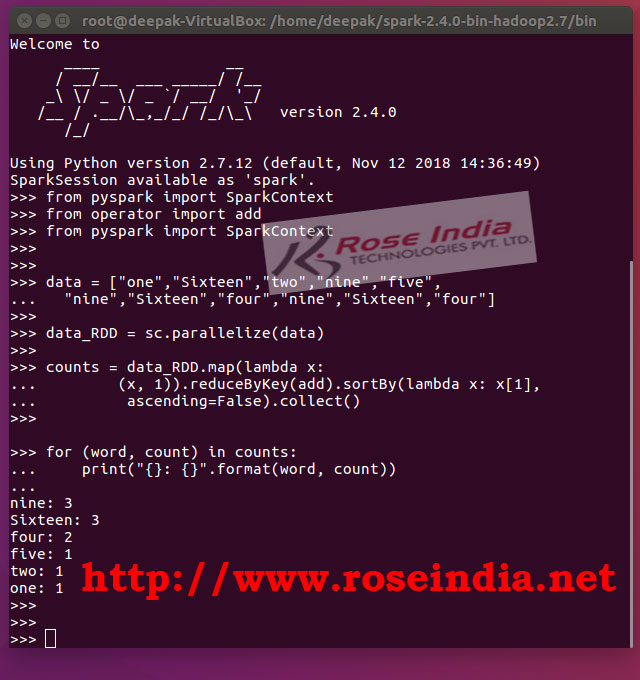# Python Spark Map function example

In this tutorial we will teach you to use the Map function of PySpark to write code in Python. Python Spark Map function allows developers to read each element of RDD and perform some processing.## Python Spark Map function example - Writing word count example with Map function

In this example program we are going to learn about the map() function of PySpark RDD. We will use this function in a word count program which counts the number of each unique word in the Spark RDD. This type of program is very useful in text processing and machine learning application where lots of text is being processed.

The map() function is transformation function in RDD which applies a given function to each element of RDD and then produces a new RDD. In Spark the RDD Data structure is used in many ways to process data. A very simple example is to create RDD from list or from file and then apply a function on the each line of data to generate a new RDD. In this word count we are simply counting number of occurrences of each word in the data.

If you want to learn data structures in Spark then check our tutorial: Apache Spark data structures. If you are beginner in Spark Programming then you should learn the Apache Spark data structures.

### Syntax of map() function

map(function) : It returns RDD which is a result of function applied on the each element of RDD. Here developers can write own function to manipulate the data as per business processing needs.

The map() transformation is most commonly used transformation in Apache Spark framework. The map() transformation is design to take a function and then it applies this function to each element in the RDD. The applied function returns a new RDD and this way new RDD is generated with the transformed data.

Here is example program of map() function which is used for creating word count example in PySpark:

```from pyspark import SparkContext
from pyspark import SparkContext

data = ["one","Sixteen","two","nine","five",
"nine","Sixteen","four","nine","Sixteen","four"]

data_RDD = sc.parallelize(data)

counts = data_RDD.map(lambda x:
ascending=False).collect()

for (word, count) in counts:
print("{}: {}".format(word, count))

```

You can run this example in PySpark shell to view the output. Here is the screen shot of PySpark shell:If you run above example it will show you count of each word shorted by count. There are many use cases of map function in PySpark. Its one of the most used method in PySpark. In this tutorial we have seen the use of map() function with simple example code.

We have large number of Apache Spark tutorials. Check these links: